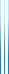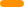# PublicationsPapers

## Shrinkage under random projections and cubic formula lower bounds for AC^0

Yuval Filmus, Or Meir and Avishay Tal
ITCS 2021

Lower bounds on concrete functions are very hard to prove. The best known lower bound on the circuit complexity of any concrete function is only linear. In contrast, we have a nearly cubic bound on the formula complexity of a concrete function, namely Andreev’s function. This lower bound is proved via shrinkage, and was first obtained in this strong form by Håstad. Later on, Avishay Tal reproved the result using different techniques, shaving some lower order factors.

Shrinkage is the phenomenon in which a de Morgan formula shrinks by a factor of $O(p^2)$ (in expectation and with high probability) when subjected to a random restriction which leaves only a $p$-fraction of its values alive. In this regard, shrinkage is similar to the switching lemma, which is about simplification of DNFs and CNFs. However, in contrast to the switching lemma, which is known to hold for a variety of distributions, shrinkage has only been considered for standard random restrictions.

In this paper we prove a more general switching lemma, which works for two types of random projections (generalizing random restrictions) which we call fixing and hiding. As an application, we prove a (nearly) cubic formula lower bound for an Andreev-like function in $\mathsf{AC^0}$. Using a different kind of random restriction is necessary in this case, since standard random restrictions are known to drastically simplify functions in $\mathsf{AC^0}$, by the switching lemma. Our proof closely follows Håstad’s, and could serve as an exposition of his proof.

## BibTeX

@inproceedings{FMT2021,
title = {Shrinkage under random projections and cubic formula lower bounds for {$mathit{AC}^0$}},
author = {Yuval Filmus and Or Meir and Avishay Tal},
booktitle = {12th Innovations in Theoretical Computer Science Conference (ITCS 2021)},
year = {2021}}copy to clipboard